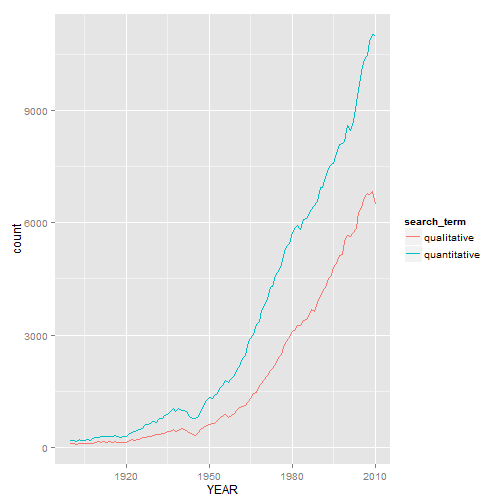# This is a tutorial on how to download data from Jstor.

Jstor is a repository of academic journals. Recently, Jstor has allowed people to search and download bibliometric data.

One aspect of the bibliometric data available for download are counts of words. Jstor has indexed words that appear in its database of articles.

It is possible to search for, download, and analyze these data.

This tutorial will demonstrate how to accomplish this with R. In particular, we will search for the terms 'quantitative' and 'qualitative' in academic journal articles from 1900 to 2010. We will then graph how many times these two terms have been mentioned in Jstor over time.

## Create a dataframe to house the data.

We will first create a dataframe to house the data. We will start off with a dataframe that has one column, and 111 rows - equal to the number of years from 1900 to 2010.

``````data <- as.data.frame(matrix(ncol=1, nrow=111))
``````

## Create a column of years.

Next, we will list out the years in sequence, and rename the column to “YEAR”

``````data\$V1 <- seq(from=1900, to=2010)

names(data)[names(data)=="V1"] <- "YEAR"
``````

## Specify the terms to search for in Jstor.

``````search_terms <- c("quantitative", "qualitative")
``````

## Loop through those search terms and pull in Jstor data.

``````# Create a loop
for(i in 1:length(search_terms)){

# Get each search term and store those in objects
term <- search_terms[i]

# Download the data, using a URL to query Jstor
x <- paste("http://dfr.jstor.org/fsearch/csv?cs=any%3A%22", term,
"%22%7Cty%3Afla%5E1.0&fs=tym1&view=chart&&csv=current_year", sep="")

# Read in resulting CSV file
temp <- read.csv(x)

# Merge Jstor data with dataframe created step 1, matching by years
data <- merge(data, temp, by ="YEAR", all.x=TRUE)

# Remove temporary dataframe
rm(temp)

# Reaname column by search term
colname <- paste(term, sep="")

# Rename added column with ID
names(data)[names(data)=="ARTICLE_COUNT"] <- colname

}
``````

## Inspect the data frame downloaded.

Look at the top six rows and the last six rows.

``````head(data)
``````
``````##   YEAR quantitative qualitative
## 1 1900          178         101
## 2 1901          181          98
## 3 1902          170          89
## 4 1903          202          97
## 5 1904          188         111
## 6 1905          185          99
``````
``````tail(data)
``````
``````##     YEAR quantitative qualitative
## 106 2005        10035        6410
## 107 2006        10363        6686
## 108 2007        10460        6783
## 109 2008        10844        6763
## 110 2009        11021        6833
## 111 2010        11011        6496
``````

## Reshape the data to put it in a graph friendly (long) format.

``````data_long <- reshape(data,
varying = c("quantitative", "qualitative"),
v.names = "count",
timevar = "search_term",
times = c("quantitative", "qualitative"),
direction = "long")
``````

## Graph the data to observe trends over time.

As you can see from the graph below, academic journal articles have mentioned “quantitative” more frequently than “qualitative” throughout years, but the disparity in mentions increases over time.``````library(ggplot2)

p <- ggplot(data_long, aes(x=YEAR, y=count, group=search_term))

p +  geom_line(aes(colour = search_term))
``````